This lesson is being piloted (Beta version)

# Handling Spatial Projection & CRS in R

## Overview

Teaching: 40 min
Exercises: 20 min
Questions
• What do I do when vector data don’t line up?

Objectives
• Plot vector objects with different CRSs in the same plot.

We will continue to work with the `sf` and the `ggplot2` packages that you have worked with in previous lessons.

``````#library(rgdal)
library(sf)
library(ggplot2)
``````

## Things You’ll Need To Complete This Episode

See the lesson homepage for detailed information about the software, data, and other prerequisites you will need to work through the examples in this episode.

In an earlier episode we learned how to handle a situation where you have two different files with raster data in different projections. Now we will apply those same principles to working with vector data.

We will create a base map of our study site using United States state and country boundary information accessed from the United States Census Bureau. We will learn how to map vector data that are in different CRSs and thus don’t line up on a map.

We will continue to work with one of the shapefiles that we loaded in the Open and Plot Shapefiles in R episode. If you are continuning directly from earlier lessons, you may already have that object in your environment. If not, you should load the layer now.

``````# load vector data for the HARV site
point_HARV <- st_read("data/vector/HARVtower_UTM18N.shp")
``````

## Working With Spatial Data From Different Sources

We often need to gather spatial datasets from different sources and/or data that cover different spatial extents. These data are often in different Coordinate Reference Systems (CRSs).

Some reasons for data being in different CRSs include:

1. The data are stored in a particular CRS convention used by the data provider (for example, a government agency).
2. The data are stored in a particular CRS that is customized to a region. For instance, many states in the US prefer to use a State Plane projection customized for that state.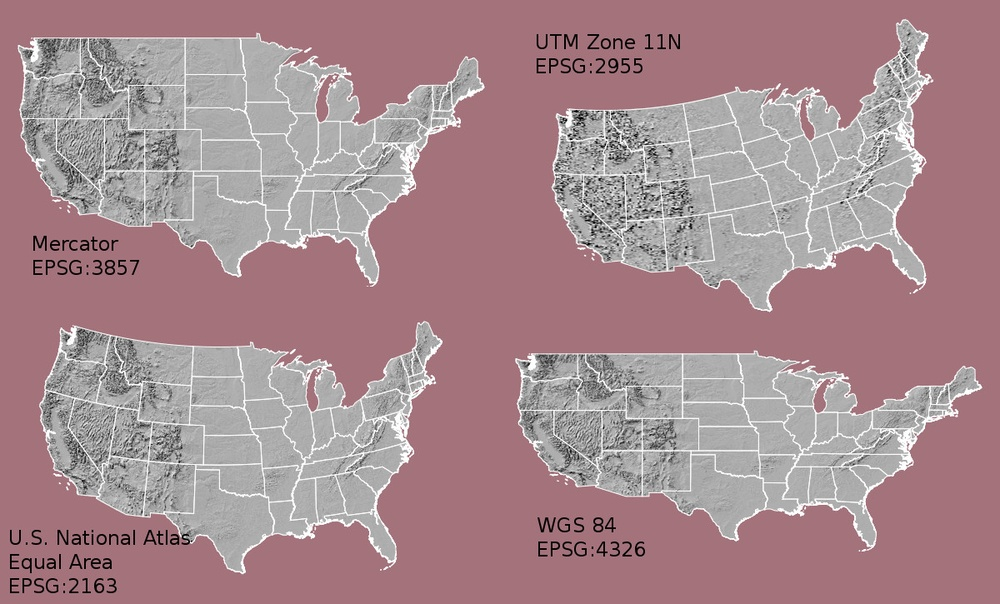Notice the differences in shape associated with each different projection. These differences are a direct result of the calculations used to “flatten” the data onto a 2-dimensional map. Often data are stored purposefully in a particular projection that optimizes the relative shape and size of surrounding geographic boundaries (states, counties, countries, etc).

In this episode we will learn how to identify and manage spatial data in different projections. We will learn how to reproject the data so that they are in the same projection to support plotting / mapping. Note that these skills are also required for any geoprocessing / spatial analysis. Data need to be in the same CRS to ensure accurate results.

## Import US Boundaries - Census Data

There are many good sources of boundary base layers that we can use to create a basemap. Some R packages even have these base layers built in to support quick and efficient mapping. In this episode, we will use boundary layers for the contiguous United States, provided by the United States Census Bureau. It is useful to have shapefiles to work with because we can add additional attributes to them if need be - for project specific mapping.

## Read US Boundary File

We will use the `st_read()` function to import the `US-State-Boundaries-Census-2014` layer into R. This layer contains the boundaries of all contiguous states in the U.S. Please note that these data have been modified and reprojected from the original data downloaded from the Census website to support the learning goals of this episode.

``````state_boundary_US <- st_read("data/vector/US-State-Boundaries-Census-2014.shp")
``````
``````Reading layer `US-State-Boundaries-Census-2014' from data source `/home/travis/build/UW-Madison-DataScience/r-raster-vector-geospatial/_episodes_rmd/data/vector/US-State-Boundaries-Census-2014.shp' using driver `ESRI Shapefile'
Simple feature collection with 58 features and 10 fields
geometry type:  MULTIPOLYGON
dimension:      XYZ
bbox:           xmin: -124.7258 ymin: 24.49813 xmax: -66.9499 ymax: 49.38436
z_range:        zmin: 0 zmax: 0
CRS:            4326
``````

Next, let’s plot the U.S. states data. We will use `ggplot2` for a quick plot of the vector data, with an informative title.

``````ggplot() +
geom_sf(data = state_boundary_US) +
ggtitle("Map of Contiguous US State Boundaries") +
coord_sf()
``````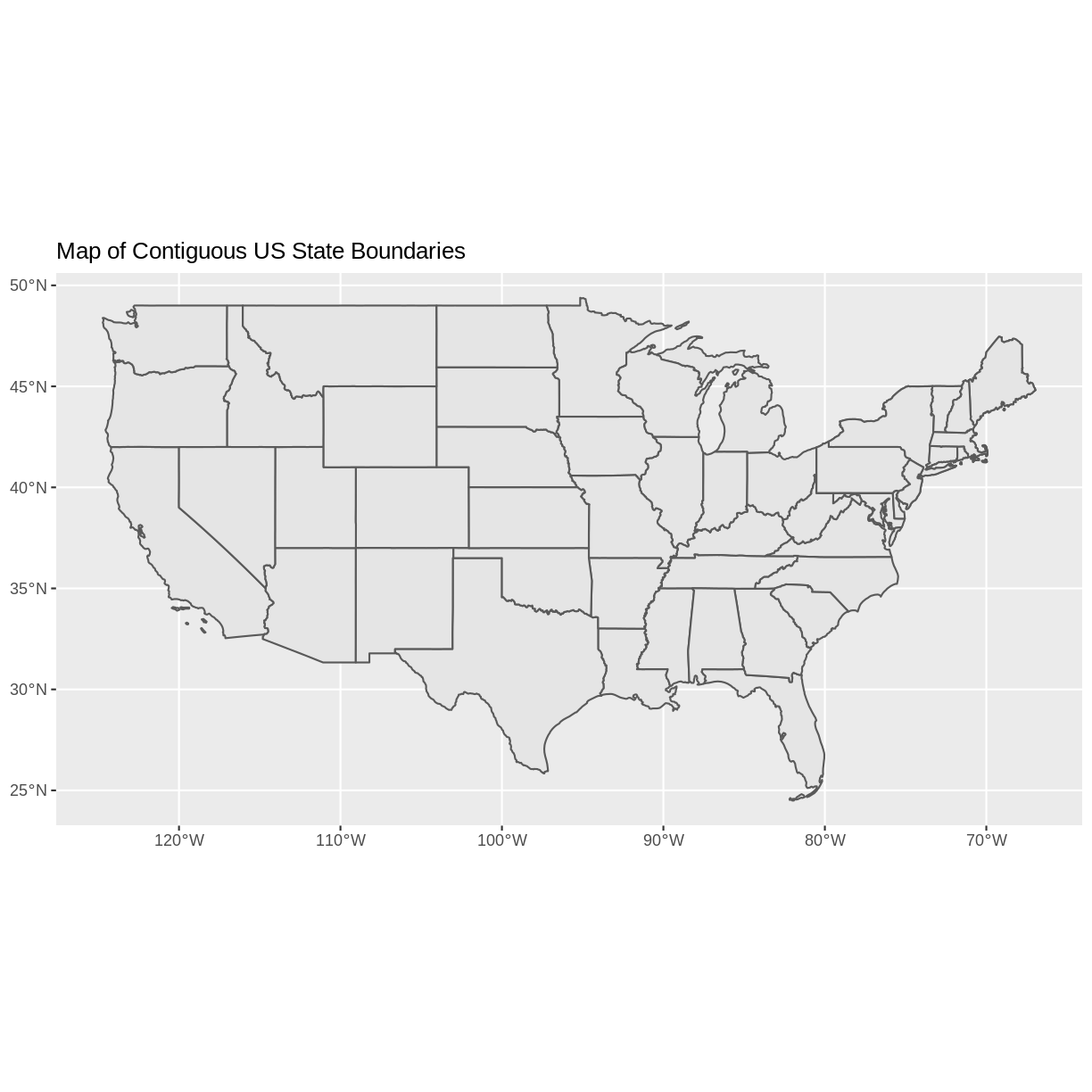## U.S. Boundary Layer

We can add a boundary layer of the United States to our map - to make it look nicer. We will import `US-Boundary-Dissolved-States`. This vector layer is the outline of the US contiguous states. Is it called dissolved because the state boundaries have been removed, or dissolved, leaving just the outline. While dissolve may seem like an unusual term, it is a common geospatial task.

``````country_boundary_US <- st_read("data/vector/US-Boundary-Dissolved-States.shp")
``````
``````Reading layer `US-Boundary-Dissolved-States' from data source `/home/travis/build/UW-Madison-DataScience/r-raster-vector-geospatial/_episodes_rmd/data/vector/US-Boundary-Dissolved-States.shp' using driver `ESRI Shapefile'
Simple feature collection with 1 feature and 9 fields
geometry type:  MULTIPOLYGON
dimension:      XYZ
bbox:           xmin: -124.7258 ymin: 24.49813 xmax: -66.9499 ymax: 49.38436
z_range:        zmin: 0 zmax: 0
CRS:            4326
``````

We can add the boundary to our figure by adding a layer to the `ggplot()` block. If we specify a thicker line width using `size = 2` for the border layer, it will make our map pop! We will also manually set the colors of the state boundaries and country boundaries.

``````ggplot() +
geom_sf(data = country_boundary_US, size = 2) +
geom_sf(data = state_boundary_US) +
ggtitle("Map of Contiguous US State Boundaries") +
coord_sf()
``````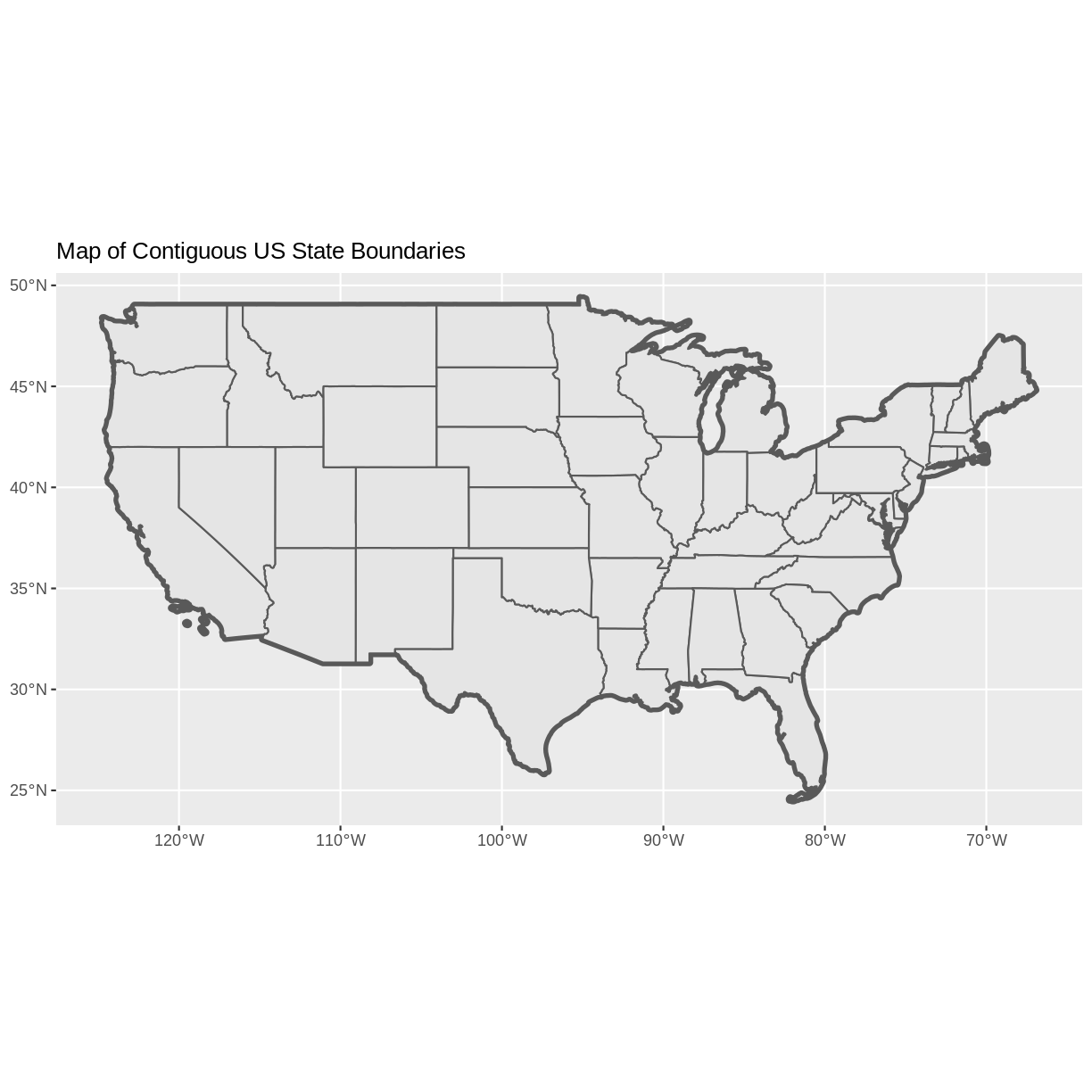Next, let’s add the location of a flux tower where our study area is in the HARV site. As we are adding these layers, take note of the CRS of each object.

First let’s look at the CRS of our tower location object. We will use the `st_crs` function and assign it to an object. This will make it easier for us to compare with the other vector data. As we saw in an earlier episode, the project string gives us a lot of the project information that we need.

``````point_HARV_crs <- st_crs(point_HARV)
point_HARV_crs\$proj4string
``````
`````` "+proj=utm +zone=18 +datum=WGS84 +units=m +no_defs "
``````

Our project string for `point_HARV` specifies the UTM projection as follows:

`+proj=utm +zone=18 +datum=WGS84 +units=m +no_defs`

• proj=utm: the projection is UTM, UTM has several zones.
• zone=18: the zone is 18
• datum=WGS84: the datum WGS84 (the datum refers to the 0,0 reference for the coordinate system used in the projection)
• units=m: the units for the coordinates are in METERS.

Note that the `zone` is unique to the UTM projection. Not all CRSs will have a zone.

Let’s check the CRS of our state and country boundary objects:

``````st_crs(state_boundary_US)\$proj4string
``````
`````` "+proj=longlat +datum=WGS84 +no_defs "
``````
``````st_crs(country_boundary_US)\$proj4string
``````
`````` "+proj=longlat +datum=WGS84 +no_defs "
``````

Our project string for `state_boundary_US` and `country_boundary_US` specifies the lat/long projection as follows:

`+proj=longlat +datum=WGS84 +no_defs`

• proj=longlat: the data are in a geographic (latitude and longitude) coordinate system
• datum=WGS84: the datum WGS84 (the datum refers to the 0,0 reference for the coordinate system used in the projection)

Note that there are no specified units above. This is because this geographic coordinate reference system is in latitude and longitude which is most often recorded in decimal degrees.

## CRS Units - View Object Extent

From looking at the project string, we know that the HARV data and the US data are in different projections. But let’s explore the data further. Next, let’s view the extent or spatial coverage for the `point_HARV` spatial object compared to the `state_boundary_US` object.

First we’ll look at the extent for our study site:

``````st_bbox(point_HARV)
``````
``````     xmin      ymin      xmax      ymax
732183.2 4713265.0  732183.2 4713265.0
``````

And then the extent for the state boundary data.

``````st_bbox(state_boundary_US)
``````
``````      xmin       ymin       xmax       ymax
-124.72584   24.49813  -66.94989   49.38436
``````

Note the difference in the units for each object. The extent for `state_boundary_US` is in latitude and longitude which yields smaller numbers representing decimal degree units. Our tower location point is in UTM, is represented in meters.

## Reproject Vector Data or No?

We saw in an earlier episode that when working with raster data in different CRSs, we needed to convert all objects to the same CRS. We can do the same thing with our vector data - however, we don’t need to! When using the `ggplot2` package, `ggplot` automatically converts all objects to the same CRS before plotting. This means we can plot our three data sets together without doing any conversion:

``````ggplot() +
geom_sf(data = country_boundary_US, size = 2) +
geom_sf(data = state_boundary_US) +
geom_sf(data = point_HARV, shape = 19, color = "purple") +
ggtitle("Map of Contiguous US State Boundaries") +
coord_sf()
``````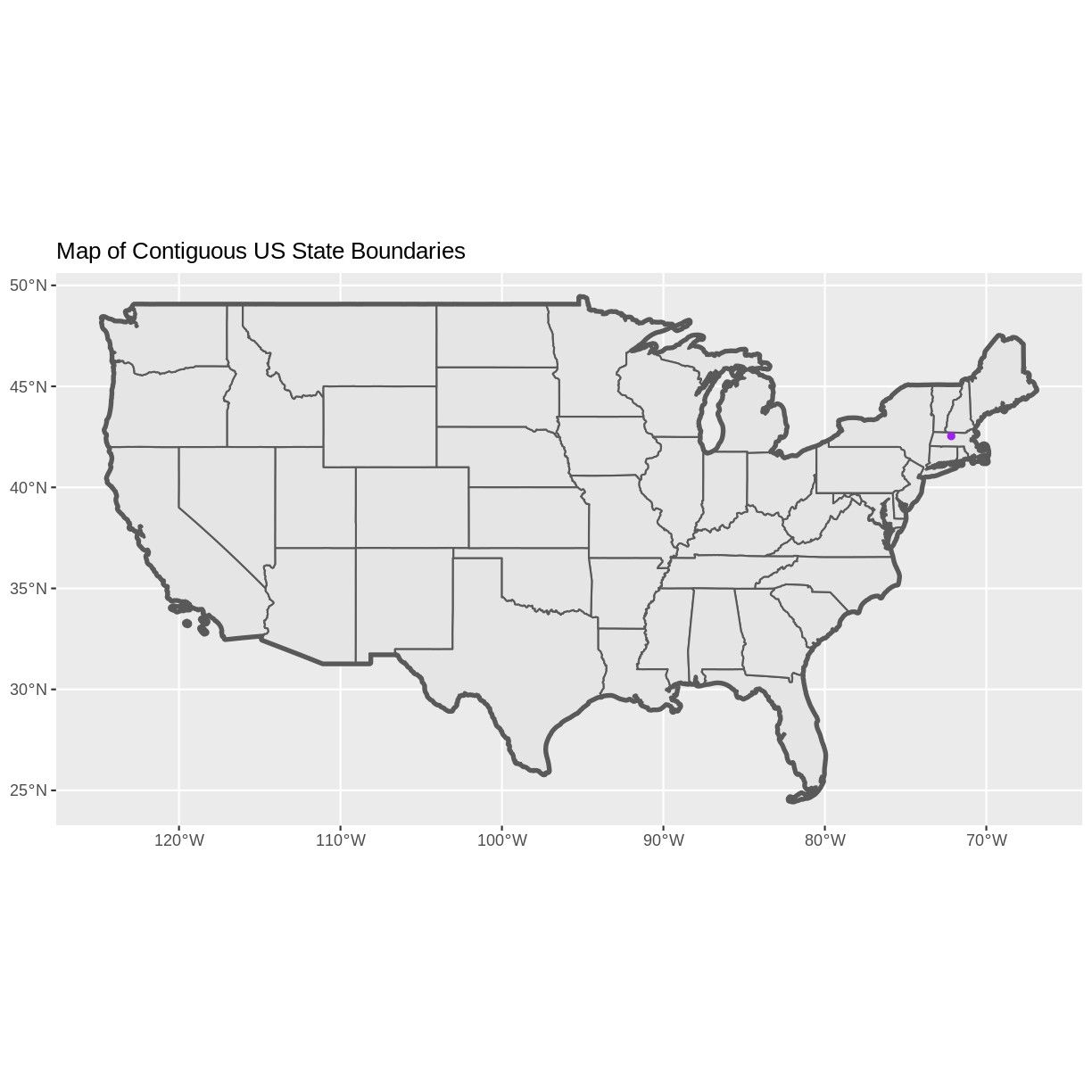## Challenge - Plot Multiple Layers of Spatial Data

Create a map of the North Eastern United States as follows:

1. Import and plot `Boundary-US-State-NEast.shp`. Adjust line width as necessary.
2. Layer the Fisher Tower (in the NEON Harvard Forest site) point location `point_HARV` onto the plot.
3. Add a title.
4. Add a legend that shows both the state boundary (as a line) and the Tower location point.

## Answers

``````NE.States.Boundary.US <- st_read("data/vector/Boundary-US-State-NEast.shp")
``````
``````Reading layer `Boundary-US-State-NEast' from data source `/home/travis/build/UW-Madison-DataScience/r-raster-vector-geospatial/_episodes_rmd/data/vector/Boundary-US-State-NEast.shp' using driver `ESRI Shapefile'
Simple feature collection with 12 features and 9 fields
geometry type:  MULTIPOLYGON
dimension:      XYZ
bbox:           xmin: -80.51989 ymin: 37.91685 xmax: -66.9499 ymax: 47.45716
z_range:        zmin: 0 zmax: 0
CRS:            4326
``````
``````ggplot() +
geom_sf(data = NE.States.Boundary.US, aes(color ="color"), show.legend = "line") +
scale_color_manual(name = "", labels = "State Boundary", values = c("color" = "gray18")) +
geom_sf(data = point_HARV, aes(shape = "shape"), color = "purple") +
scale_shape_manual(name = "", labels = "Fisher Tower", values = c("shape" = 19)) +
ggtitle("Fisher Tower location") +
theme(legend.background = element_rect(color = NA)) +
coord_sf()
``````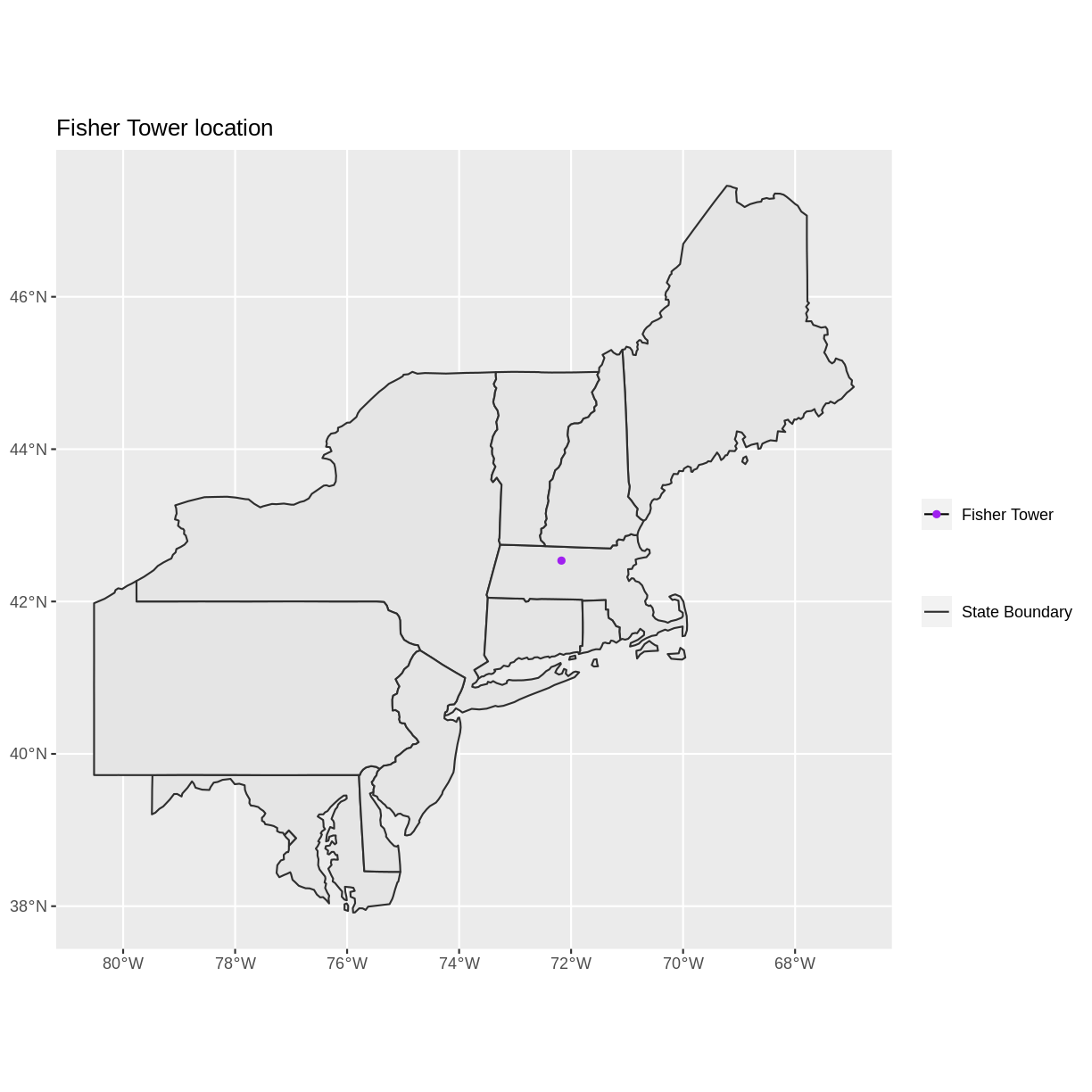## Key Points

• `ggplot2` automatically converts all objects in a plot to the same CRS.

• Still be aware of the CRS and extent for each object.